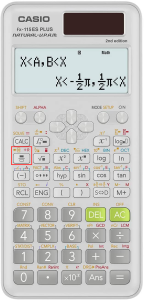# How To Do Fractions On A Scientific Calculator

While scientific calculators are designed to help solve complex engineering, physics, math, and chemistry problems, like business calculators, they display fractions as decimals by default.

This means if you enter a fraction like ¾ on the calculator, the display will read 0.75.

However, most scientific calculators have a feature that enables you to display fractions as a result without making the conversion yourself.

Perhaps the best thing about the feature is that you can also enter complex or improper fractions into the calculator and have it simplify them for you.

Here’s a quick guide to doing fractions on your scientific calculator.

## How to Do Fractions on A Scientific Calculator

Scientific calculators come loaded with a special mode called the “MATH mode.” To use your calculator for fractions, this is the mode you must use.

### Step #1: Put Your Calculator In MATH mode

To do this, you must first push the “MODE” button on the calculator to open the modes menu.

Then, you have to navigate to the “MATH” mode option using the arrow keys. When you activate the mode, the calculator will display the word “MATH” on top of the screen.

There is a chance your scientific calculator doesn’t have this function. You can proceed to the next section to find a workaround to do fractions with such a calculator.

### Step #2: Press the Fraction Button

After you toggle the MATH mode, you will need to press the fraction button to input the numbers in that format and use the fraction calculator.

Most scientific calculators represent the fraction button as a black box over a white box.However, some calculators have “x/y” or “b/c” stamped on the fraction key instead.

When you press this button, the fraction template will appear on the screen. The template will have two blank boxes, one on top of the other, separated by a horizontal line.

Some calculators separate the two boxes with an inverted “L” instead of a horizontal line.

If your calculator doesn’t have a MATH mode but has a fraction button, try pressing the fraction button to make the template appear directly.

Note: If you want to enter a mixed fraction, you can push the SHIFT key on your calculator before pressing the fraction button. Doing this will insert a third box in the template, allowing you to enter a number there.

### Step #3: Enter the Numerator

Now that you have a template on your screen, enter the numerator of your fraction in the top box using the keypad.

The calculator’s cursor will start there, so you don’t have to do any navigating with the arrow keys.

### Step #4: Navigate to the Denominator

After entering the numerator, you must enter the denominator of the fractional.

Before you enter the number, you will need to navigate to the lower box of the template. To do this, press the down arrow on your calculator. This will move the cursor to the lower box.

If your scientific calculator has an “L” instead of a horizontal line separating the two boxes, you may need to hit the right arrow instead of the down arrow to move the cursor.

### Step #5: Enter the Denominator

Now that your cursor is correctly positioned in the lower box, use the keypad to enter the denominator.

Before you hit the “equals” button, look at the calculator’s display and ensure that your fraction looks right. If you’ve accidentally entered a wrong number, you can use the arrow keys and the delete key to remove the number and enter the right one.

### Step #6: Hit the Equals Button

Hitting the equals button will simplify a complex fraction or give you the results of your regular fraction, depending on what you’ve entered.

## Calculating Fractions Without the Fraction Key

If your calculator doesn’t have the fraction key or MATH mode, there is a workaround you can use to manipulate fractions.

### Write the Fraction as A Decimal And Convert

If you’re unable to display fractions on your calculator, remember that you can still enter them.

To write a fraction as a decimal, enter the numerator with the keypad, hit the division key, then enter the denominator. Hitting the “equals” button will return the answer as a decimal.

While your calculator may not be able to convert the decimal into a fraction for you, it can help you do it with a pencil and paper.

Let’s say you want to show 0.5714 as a fraction. You could write it as 5714/10000, but you will likely want to reduce the fraction down to something a lot simpler.

To do this, multiply the decimal number with a possible denominator. This will give you a numerator for the fraction. You will need to do some guesswork, and this method of working is based on trial and error.

For instance, let’s try multiplying the decimal with 7. 7 x 0.5714 = 3.998, which is close enough to 4.

So, on paper, you can write the fraction 4/7 to represent 0.5714.

## Conclusion

Scientific calculators are some of the most powerful tools students have in their arsenal when tackling complex problems of all sorts.

And now that you know how to use the scientific calculator to do fractions, you can use it to its full potential.

You don’t need to buy a new calculator if yours doesn’t have the MATH mode or the function key. You can convert your decimal into a fraction with the help of your calculator using the steps mentioned above.

We have various comprehensive calculators that you can use online for free. You can choose from t-test calculator, graphing, matrix, the standard deviation to statistics, and scientific calculators. Check it here